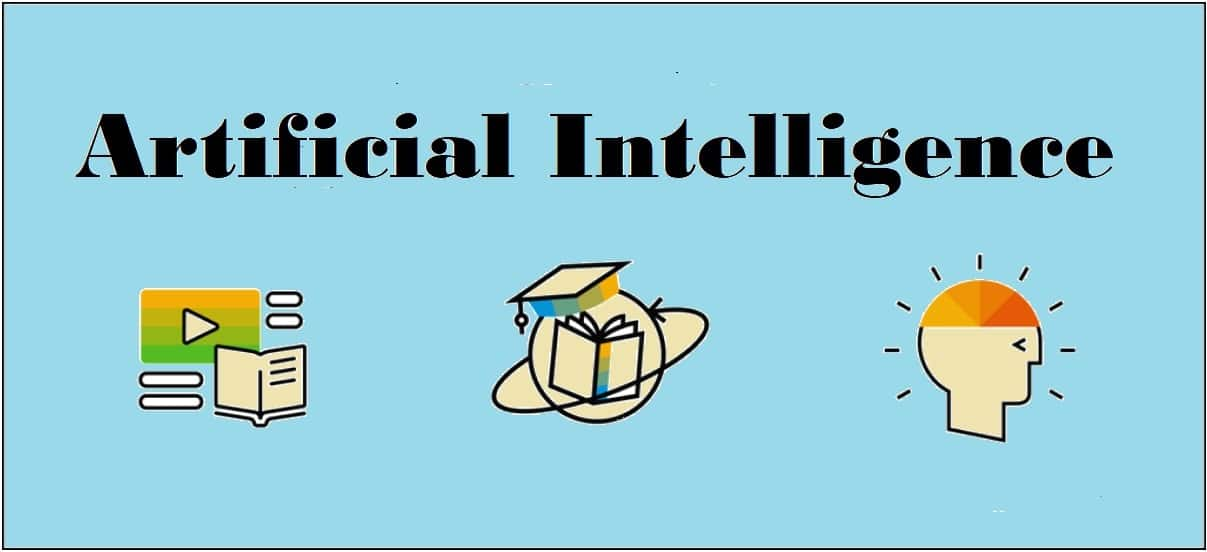## Deep Learning

Deep learning emerged from a decade’s explosive computational growth as a serious contender in the field. Thus, deep learning is a particular kind of machine learning whose algorithms are inspired by the structure and function of human brain.

### Machine Learning vs Deep Learning

Deep learning is the most powerful machine learning technique these days. It is so powerful because they learn the best way to represent the problem while learning how to solve the problem. A comparison of Deep learning and Machine learning is given below −

### Data Dependency

The first point of difference is based upon the performance of DL and ML when the scale of data increases. When the data is large, deep learning algorithms perform very well.

### Machine Dependency

Deep learning algorithms need high-end machines to work perfectly. On the other hand, machine learning algorithms can work on low-end machines too.

### Feature Extraction

Deep learning algorithms can extract high level features and try to learn from the same too. On the other hand, an expert is required to identify most of the features extracted by machine learning.

### Time of Execution

Execution time depends upon the numerous parameters used in an algorithm. Deep learning has more parameters than machine learning algorithms. Hence, the execution time of DL algorithms, specially the training time, is much more than ML algorithms. But the testing time of DL algorithms is less than ML algorithms.

### Approach to Problem Solving

Deep learning solves the problem end-to-end while machine learning uses the traditional way of solving the problem i.e. by breaking down it into parts.

[wpsbx_html_block id=1891]

### Convolutional Neural Network (CNN)

Convolutional neural networks are the same as ordinary neural networks because they are also made up of neurons that have learnable weights and biases. Ordinary neural networks ignore the structure of input data and all the data is converted into 1-D array before feeding it into the network. This process suits the regular data, however if the data contains images, the process may be cumbersome.

CNN solves this problem easily. It takes the 2D structure of the images into account when they process them, which allows them to extract the properties specific to images. In this way, the main goal of CNNs is to go from the raw image data in the input layer to the correct class in the output layer. The only difference between an ordinary NNs and CNNs is in the treatment of input data and in the type of layers.

### Architecture Overview of CNNs

Architecturally, the ordinary neural networks receive an input and transform it through a series of hidden layer. Every layer is connected to the other layer with the help of neurons. The main disadvantage of ordinary neural networks is that they do not scale well to full images.

The architecture of CNNs have neurons arranged in 3 dimensions called width, height and depth. Each neuron in the current layer is connected to a small patch of the output from the previous layer. It is similar to overlaying a 𝑵×𝑵 filter on the input image. It uses M filters to be sure about getting all the details. These M filters are feature extractors which extract features like edges, corners, etc.

### Layers used to construct CNNs

Following layers are used to construct CNNs −

• Input Layer − It takes the raw image data as it is.
• Convolutional Layer − This layer is the core building block of CNNs that does most of the computations. This layer computes the convolutions between the neurons and the various patches in the input.
• Rectified Linear Unit Layer − It applies an activation function to the output of the previous layer. It adds non-linearity to the network so that it can generalize well to any type of function.
• Pooling Layer − Pooling helps us to keep only the important parts as we progress in the network. Pooling layer operates independently on every depth slice of the input and resizes it spatially. It uses the MAX function.
• Fully Connected layer/Output layer − This layer computes the output scores in the last layer. The resulting output is of the size 𝟏×𝟏×𝑳 , where L is the number training dataset classes.

### Installing Useful Python Packages

You can use Keras, which is an high level neural networks API, written in Python and capable of running on top of TensorFlow, CNTK or Theno. It is compatible with Python 2.7-3.6. You can learn more about it from https://keras.io/.

Use the following commands to install keras −

pip install keras


On conda environment, you can use the following command −

conda install –c conda-forge keras


### Building Linear Regressor using ANN

In this section, you will learn how to build a linear regressor using artificial neural networks. You can use KerasRegressor to achieve this. In this example, we are using the Boston house price dataset with 13 numerical for properties in Boston. The Python code for the same is shown here −

Import all the required packages as shown −

import numpy
import pandas
from keras.models import Sequential
from keras.layers import Dense
from keras.wrappers.scikit_learn import KerasRegressor
from sklearn.model_selection import cross_val_score
from sklearn.model_selection import KFold

Now, load our dataset which is saved in local directory.

dataframe = pandas.read_csv("/Usrrs/admin/data.csv", delim_whitespace = True, header = None)
dataset = dataframe.values

Now, divide the data into input and output variables i.e. X and Y −

X = dataset[:,0:13]
Y = dataset[:,13]

Since we use baseline neural networks, define the model −

def baseline_model():

Now, create the model as follows −

model_regressor = Sequential()
model_regressor.add(Dense(13, input_dim = 13, kernel_initializer = 'normal',
activation = 'relu'))
model_regressor.add(Dense(1, kernel_initializer = 'normal'))

Next, compile the model −

model_regressor.compile(loss='mean_squared_error', optimizer='adam')
return model_regressor

Now, fix the random seed for reproducibility as follows −

seed = 7
numpy.random.seed(seed)

The Keras wrapper object for use in scikit-learn as a regression estimator is called KerasRegressor. In this section, we shall evaluate this model with standardize data set.

estimator = KerasRegressor(build_fn = baseline_model, nb_epoch = 100, batch_size = 5, verbose = 0)
kfold = KFold(n_splits = 10, random_state = seed)
baseline_result = cross_val_score(estimator, X, Y, cv = kfold)
print("Baseline: %.2f (%.2f) MSE" % (Baseline_result.mean(),Baseline_result.std()))

The output of the code shown above would be the estimate of the model’s performance on the problem for unseen data. It will be the mean squared error, including the average and standard deviation across all 10 folds of the cross validation evaluation.

### Image Classifier: An Application of Deep Learning

Convolutional Neural Networks (CNNs) solve an image classification problem, that is to which class the input image belongs to. You can use Keras deep learning library. Note that we are using the training and testing data set of images of cats and dogs from following link https://www.kaggle.com/c/dogs-vs-cats/data.

Import the important keras libraries and packages as shown −

The following package called sequential will initialize the neural networks as sequential network.

from keras.models import Sequential

The following package called Conv2D is used to perform the convolution operation, the first step of CNN.

from keras.layers import Conv2D

The following package called MaxPoling2D is used to perform the pooling operation, the second step of CNN.

from keras.layers import MaxPooling2D

The following package called Flatten is the process of converting all the resultant 2D arrays into a single long continuous linear vector.

from keras.layers import Flatten

The following package called Dense is used to perform the full connection of the neural network, the fourth step of CNN.

from keras.layers import Dense

Now, create an object of the sequential class.

S_classifier = Sequential()

Now, next step is coding the convolution part.

S_classifier.add(Conv2D(32, (3, 3), input_shape = (64, 64, 3), activation = 'relu'))

Here relu is the rectifier function.

Now, the next step of CNN is the pooling operation on the resultant feature maps after convolution part.

S-classifier.add(MaxPooling2D(pool_size = (2, 2)))

Now, convert all the pooled images into a continuous vector by using flattering −

S_classifier.add(Flatten())

Next, create a fully connected layer.

S_classifier.add(Dense(units = 128, activation = 'relu'))

Here, 128 is the number of hidden units. It is a common practice to define the number of hidden units as the power of 2.

Now, initialize the output layer as follows −

S_classifier.add(Dense(units = 1, activation = 'sigmoid'))

Now, compile the CNN, we have built −

S_classifier.compile(optimizer = 'adam', loss = 'binary_crossentropy', metrics = ['accuracy'])

Here optimizer parameter is to choose the stochastic gradient descent algorithm, loss parameter is to choose the loss function and metrics parameter is to choose the performance metric.

Now, perform image augmentations and then fit the images to the neural networks −

train_datagen = ImageDataGenerator(rescale = 1./255,shear_range = 0.2,
zoom_range = 0.2,
horizontal_flip = True)
test_datagen = ImageDataGenerator(rescale = 1./255)

training_set =
(64, 64),batch_size = 32,class_mode = 'binary')

test_set =
test_datagen.flow_from_directory('test_set',target_size =
(64, 64),batch_size = 32,class_mode = 'binary')

Now, fit the data to the model we have created −

classifier.fit_generator(training_set,steps_per_epoch = 8000,epochs =
25,validation_data = test_set,validation_steps = 2000)

Here steps_per_epoch have the number of training images.

Now as the model has been trained, we can use it for prediction as follows −

from keras.preprocessing import image

target_size = (64, 64))

test_image = image.img_to_array(test_image)

test_image = np.expand_dims(test_image, axis = 0)

result = classifier.predict(test_image)

training_set.class_indices

if result == 1:
prediction = 'dog'

else:
prediction = 'cat'## Reinforcement Learning

This type of learning is used to reinforce or strengthen t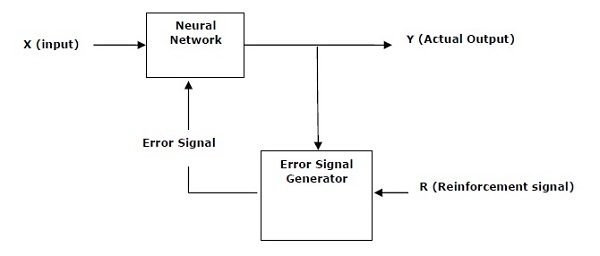he network based on critic information. That is, a network being trained under reinforcement learning, receives some feedback from the environment. However, the feedback is evaluative and not instructive as in the case of supervised learning. Based on this feedback, the network performs the adjustments of the weights to obtain better critic information in future. This learning process is similar to supervised learning but we might have very less information. The following figure gives the block diagram of reinforcement learning.

### Building Blocks: Environment and Agent

Environment and Agent are main building blocks of reinforcement learning in AI. This section discusses them in detail −

### Agent

An agent is anything that can perceive its environment through sensors and acts upon that environment through effectors.

• human agent has sensory organs such as eyes, ears, nose, tongue and skin parallel to the sensors, and other organs such as hands, legs, mouth, for effectors.
• robotic agent replaces cameras and infrared range finders for the sensors, and various motors and actuators for effectors.
• software agent has encoded bit strings as its programs and actions.

### Agent Terminology

The following terms are more frequently used in reinforcement learning in AI −

• Performance Measure of Agent − It is the criteria, which determines how successful an agent is.
• Behavior of Agent − It is the action that agent performs after any given sequence of percepts.
• Percept − It is agent’s perceptual inputs at a given instance.
• Percept Sequence − It is the history of all that an agent has perceived till date.
• Agent Function − It is a map from the precept sequence to an action.

### Environment

• Some programs operate in an entirely artificial environment confined to keyboard input, database, computer file systems and character output on a screen.
• In contrast, some software agents, such as software robots or softbots, exist in rich and unlimited softbot domains. The simulator has a very detailed, and complex environment. The software agent needs to choose from a long array of actions in real time.
• For example, a softbot designed to scan the online preferences of the customer and display interesting items to the customer works in the real as well as an artificial environment.

### Properties of Environment

The environment has multifold properties as discussed below −

• Discrete/Continuous − If there are a limited number of distinct, clearly defined, states of the environment, the environment is discrete , otherwise it is continuous. For example, chess is a discrete environment and driving is a continuous environment.
• Observable/Partially Observable − If it is possible to determine the complete state of the environment at each time point from the percepts, it is observable; otherwise it is only partially observable.
• Static/Dynamic − If the environment does not change while an agent is acting, then it is static; otherwise it is dynamic.
• Single agent/Multiple agents − The environment may contain other agents which may be of the same or different kind as that of the agent.
• Accessible/Inaccessible − If the agent’s sensory apparatus can have acc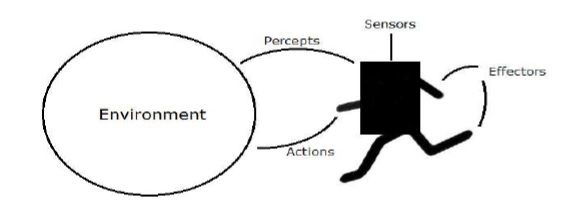ess to the complete state of the environment, then the environment is accessible to that agent; otherwise it is inaccessible.
• Deterministic/Non-deterministic − If the next state of the environment is completely determined by the current state and the actions of the agent, then the environment is deterministic; otherwise it is non-deterministic.
• Episodic/Non-episodic − In an episodic environment, each episode consists of the agent perceiving and then acting. The quality of its action depends just on the episode itself. Subsequent episodes do not depend on the actions in the previous episodes. Episodic environments are much simpler because the agent does not need to think ahead.

### Constructing an Environment with Python

For building reinforcement learning agent, we will be using the OpenAI Gym package which can be installed with the help of the following command −

pip install gym


There are various environments in OpenAI gym which can be used for various purposes. Few of them are Cartpole-v0, Hopper-v1, and MsPacman-v0. They require different engines. The detail documentation of OpenAI Gym can be found on https://gym.openai.com/docs/#environments.

The following code shows an example of Python code for cartpole-v0 environment −

import gym
env = gym.make('CartPole-v0')
env.reset()
for _ in range(1000):
env.render()
env.step(env.action_space.sample())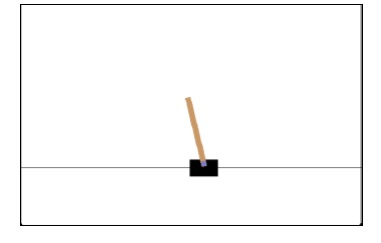You can construct other environments in a similar way.

### Constructing a learning agent with Python

For building reinforcement learning agent, we will be using the OpenAI Gym package as shown −

import gym
env = gym.make('CartPole-v0')
for _ in range(20):
observation = env.reset()
for i in range(100):
env.render()
print(observation)
action = env.action_space.sample()
observation, reward, done, info = env.step(action)
if done:
print("Episode finished after {} timesteps".format(i+1))
break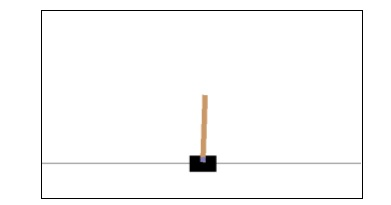Observe that the cartpole can balance itself.

[wpsbx_html_block id=1891]## Speech Recognition

Speech is the most basic means of adult human communication. The basic goal of speech processing is to provide an interaction between a human and a machine.

Speech processing system has mainly three tasks −

• First, speech recognition that allows the machine to catch the words, phrases and sentences we speak
• Second, natural language processing to allow the machine to understand what we speak, and
• Third, speech synthesis to allow the machine to speak.

This chapter focuses on speech recognition, the process of understanding the words that are spoken by human beings. Remember that the speech signals are captured with the help of a microphone and then it has to be understood by the system.

### Building a Speech Recognizer

Speech Recognition or Automatic Speech Recognition (ASR) is the center of attention for AI projects like robotics. Without ASR, it is not possible to imagine a cognitive robot interacting with a human. However, it is not quite easy to build a speech recognizer.

### Difficulties in developing a speech recognition system

Developing a high quality speech recognition system is really a difficult problem. The difficulty of speech recognition technology can be broadly characterized along a number of dimensions as discussed below −

• Size of the vocabulary − Size of the vocabulary impacts the ease of developing an ASR. Consider the following sizes of vocabulary for a better understanding.
• A small size vocabulary consists of 2-100 words, for example, as in a voice-menu system
• A medium size vocabulary consists of several 100s to 1,000s of words, for example, as in a database-retrieval task
• A large size vocabulary consists of several 10,000s of words, as in a general dictation task.

Note that, the larger the size of vocabulary, the harder it is to perform recognition.

• Channel characteristics − Channel quality is also an important dimension. For example, human speech contains high bandwidth with full frequency range, while a telephone speech consists of low bandwidth with limited frequency range. Note that it is harder in the latter.
• Speaking mode − Ease of developing an ASR also depends on the speaking mode, that is whether the speech is in isolated word mode, or connected word mode, or in a continuous speech mode. Note that a continuous speech is harder to recognize.
• Speaking style − A read speech may be in a formal style, or spontaneous and conversational with casual style. The latter is harder to recognize.
• Speaker dependency − Speech can be speaker dependent, speaker adaptive, or speaker independent. A speaker independent is the hardest to build.
• Type of noise − Noise is another factor to consider while developing an ASR. Signal to noise ratio may be in various ranges, depending on the acoustic environment that observes less versus more background noise −
• If the signal to noise ratio is greater than 30dB, it is considered as high range
• If the signal to noise ratio lies between 30dB to 10db, it is considered as medium SNR
• If the signal to noise ratio is lesser than 10dB, it is considered as low range

For example, the type of background noise such as stationary, non-human noise, background speech and crosstalk by other speakers also contributes to the difficulty of the problem.

• Microphone characteristics − The quality of microphone may be good, average, or below average. Also, the distance between mouth and micro-phone can vary. These factors also should be considered for recognition systems.

Despite these difficulties, researchers worked a lot on various aspects of speech such as understanding the speech signal, the speaker, and identifying the accents.

You will have to follow the steps given below to build a speech recognizer:

### Visualizing Audio Signals:

Reading from a File and Working on it. This is the first step in building speech recognition system as it gives an understanding of how an audio signal is structured. Some common steps that can be followed to work with audio signals are as follows −

### Recording

When you have to read the audio signal from a file, then record it using a microphone, at first.

### Sampling

When recording with microphone, the signals are stored in a digitized form. But to work upon it, the machine needs them in the discrete numeric form. Hence, we should perform sampling at a certain frequency and convert the signal into the discrete numerical form. Choosing the high frequency for sampling implies that when humans listen to the signal, they feel it as a continuous audio signal.

### Example

The following example shows a stepwise approach to analyze an audio signal, using Python, which is stored in a file. The frequency of this audio signal is 44,100 HZ.

Import the necessary packages as shown here −

import numpy as np
import matplotlib.pyplot as plt
from scipy.io import wavfile

Now, read the stored audio file. It will return two values: the sampling frequency and the audio signal. Provide the path of the audio file where it is stored, as shown here −

frequency_sampling, audio_signal = wavfile.read("/Users/admin/audio_file.wav")

Display the parameters like sampling frequency of the audio signal, data type of signal and its duration, using the commands shown −

print('\nSignal shape:', audio_signal.shape)
print('Signal Datatype:', audio_signal.dtype)
print('Signal duration:', round(audio_signal.shape /
float(frequency_sampling), 2), 'seconds')

This step involves normalizing the signal as shown below −

audio_signal = audio_signal / np.power(2, 15)

In this step, we are extracting the first 100 values from this signal to visualize. Use the following commands for this purpose −

audio_signal = audio_signal [:100]
time_axis = 1000 * np.arange(0, len(signal), 1) / float(frequency_sampling)


Now, visualize the signal using the commands given below −

plt.plot(time_axis, signal, color='blue')
plt.xlabel('Time (milliseconds)')
plt.ylabel('Amplitude')
plt.title('Input audio signal')
plt.show()

You would be able to see an output graph and data extracted for the above audio signal as shown in the image here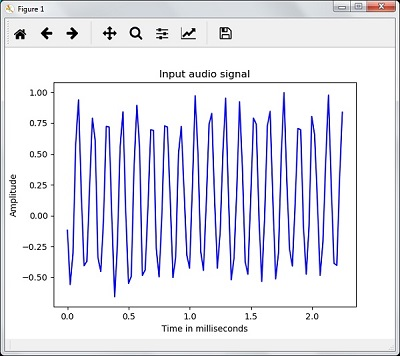Signal shape: (132300,)
Signal Datatype: int16
Signal duration: 3.0 seconds

### Characterizing the Audio Signal: Transforming to Frequency Domain

Characterizing an audio signal involves converting the time domain signal into frequency domain, and understanding its frequency components, by. This is an important step because it gives a lot of information about the signal. You can use a mathematical tool like Fourier Transform to perform this transformation.

### Example

The following example shows, step-by-step, how to characterize the signal, using Python, which is stored in a file. Note that here we are using Fourier Transform mathematical tool to convert it into frequency domain.

Import the necessary packages, as shown here −

import numpy as np
import matplotlib.pyplot as plt
from scipy.io import wavfile

Now, read the stored audio file. It will return two values: the sampling frequency and the the audio signal. Provide the path of the audio file where it is stored as shown in the command here −

frequency_sampling, audio_signal = wavfile.read("/Users/admin/sample.wav")


In this step, we will display the parameters like sampling frequency of the audio signal, data type of signal and its duration, using the commands given below −

print('\nSignal shape:', audio_signal.shape)
print('Signal Datatype:', audio_signal.dtype)
print('Signal duration:', round(audio_signal.shape /
float(frequency_sampling), 2), 'seconds')

In this step, we need to normalize the signal, as shown in the following command −

audio_signal = audio_signal / np.power(2, 15)


This step involves extracting the length and half length of the signal. Use the following commands for this purpose −

length_signal = len(audio_signal)
half_length = np.ceil((length_signal + 1) / 2.0).astype(np.int)


Now, we need to apply mathematics tools for transforming into frequency domain. Here we are using the Fourier Transform.

signal_frequency = np.fft.fft(audio_signal)

Now, do the normalization of frequency domain signal and square it −

signal_frequency = abs(signal_frequency[0:half_length]) / length_signal
signal_frequency **= 2

Next, extract the length and half length of the frequency transformed signal −

len_fts = len(signal_frequency)

Note that the Fourier transformed signal must be adjusted for even as well as odd case.

if length_signal % 2:
signal_frequency[1:len_fts] *= 2
else:
signal_frequency[1:len_fts-1] *= 2

Now, extract the power in decibal(dB) −

signal_power = 10 * np.log10(signal_frequency)

Adjust the frequency in kHz for X-axis −

x_axis = np.arange(0, len_half, 1) * (frequency_sampling / length_signal) / 1000.0

Now, visualize the characterization of signal as follows −

plt.figure()
plt.plot(x_axis, signal_power, color='black')
plt.xlabel('Frequency (kHz)')
plt.ylabel('Signal power (dB)')
plt.show()

You can observe the output graph of the above code as shown in the image below −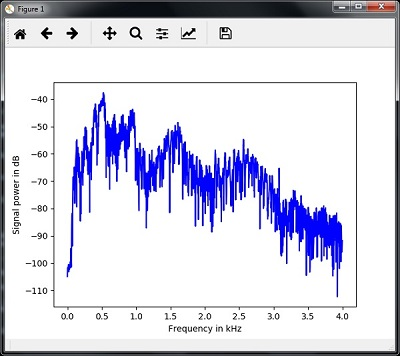### Generating Monotone Audio Signal

The two steps that you have seen till now are important to learn about signals. Now, this step will be useful if you want to generate the audio signal with some predefined parameters. Note that this step will save the audio signal in an output file.

### Example

In the following example, we are going to generate a monotone signal, using Python, which will be stored in a file. For this, you will have to take the following steps −

Import the necessary packages as shown −

import numpy as np
import matplotlib.pyplot as plt
from scipy.io.wavfile import write

Provide the file where the output file should be saved

output_file = 'audio_signal_generated.wav'


Now, specify the parameters of your choice, as shown −

duration = 4 # in seconds
frequency_sampling = 44100 # in Hz
frequency_tone = 784
min_val = -4 * np.pi
max_val = 4 * np.pi

In this step, we can generate the audio signal, as shown −

t = np.linspace(min_val, max_val, duration * frequency_sampling)
audio_signal = np.sin(2 * np.pi * tone_freq * t)

Now, save the audio file in the output file −

write(output_file, frequency_sampling, signal_scaled)


Extract the first 100 values for our graph, as shown −

audio_signal = audio_signal[:100]
time_axis = 1000 * np.arange(0, len(signal), 1) / float(sampling_freq)

Now, visualize the generated audio signal as follows −

plt.plot(time_axis, signal, color='blue')
plt.xlabel('Time in milliseconds')
plt.ylabel('Amplitude')
plt.title('Generated audio signal')
plt.show()

You can observe the plot as shown in the figure given here −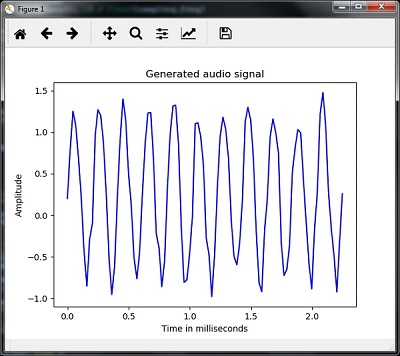### Feature Extraction from Speech

This is the most important step in building a speech recognizer because after converting the speech signal into the frequency domain, we must convert it into the usable form of feature vector. We can use different feature extraction techniques like MFCC, PLP, PLP-RASTA etc. for this purpose.

### Example

In the following example, we are going to extract the features from signal, step-by-step, using Python, by using MFCC technique.

Import the necessary packages, as shown here −

import numpy as np
import matplotlib.pyplot as plt
from scipy.io import wavfile
from python_speech_features import mfcc, logfbank

Now, read the stored audio file. It will return two values − the sampling frequency and the audio signal. Provide the path of the audio file where it is stored.

frequency_sampling, audio_signal = wavfile.read("/Users/admin/audio_file.wav")

Note that here we are taking first 15000 samples for analysis.

audio_signal = audio_signal[:15000]

Use the MFCC techniques and execute the following command to extract the MFCC features −

features_mfcc = mfcc(audio_signal, frequency_sampling)


Now, print the MFCC parameters, as shown −

print('\nMFCC:\nNumber of windows =', features_mfcc.shape)
print('Length of each feature =', features_mfcc.shape)

Now, plot and visualize the MFCC features using the commands given below −

features_mfcc = features_mfcc.T
plt.matshow(features_mfcc)
plt.title('MFCC')

In this step, we work with the filter bank features as shown −

Extract the filter bank features −

filterbank_features = logfbank(audio_signal, frequency_sampling)

Now, print the filterbank parameters.

print('\nFilter bank:\nNumber of windows =', filterbank_features.shape)
print('Length of each feature =', filterbank_features.shape)

Now, plot and visualize the filterbank features.

filterbank_features = filterbank_features.T
plt.matshow(filterbank_features)
plt.title('Filter bank')
plt.show()

As a result of the steps above, you can observe the following outputs: Figure1 for MFCC and Figure2 for Filter Bank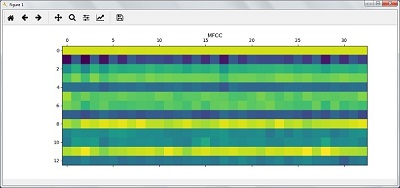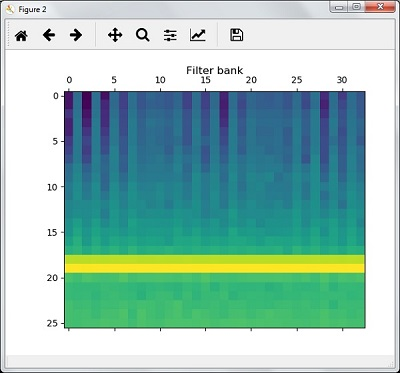### Recognition of Spoken Words

Speech recognition means that when humans are speaking, a machine understands it. Here we are using Google Speech API in Python to make it happen. We need to install the following packages for this −

• Pyaudio − It can be installed by using pip install Pyaudio command.
• SpeechRecognition − This package can be installed by using pip install SpeechRecognition.
• Google-Speech-API − It can be installed by using the command pip install google-api-python-client.

### Example

Observe the following example to understand about recognition of spoken words −

Import the necessary packages as shown −

import speech_recognition as sr

Create an object as shown below −

recording = sr.Recognizer()

Now, the Microphone() module will take the voice as input −

with sr.Microphone() as source: recording.adjust_for_ambient_noise(source)
audio = recording.listen(source)

Now google API would recognize the voice and gives the output.

try:
except Exception as e:
print(e)


You can see the following output −

Please Say Something:
You said:


For example, if you said tutorialspoint.com, then the system recognizes it correctly as follows −

tutorialspoint.com

[wpsbx_html_block id=1891]## Unsupervised Learning Clustering

Unsupervised machine learning algorithms do not have any supervisor to provide any sort of guidance. That is why they are closely aligned with what some call true artificial intelligence. In unsupervised learning, there would be no correct answer and no teacher for the guidance. Algorithms need to discover the interesting pattern in data for learning.

### Clustering:

Basically, it is a type of unsupervised learning method and a common technique for statistical data analysis used in many fields. Clustering mainly is a task of dividing the set of observations into subsets, called clusters, in such a way that observations in the same cluster are similar in one sense and they are dissimilar to the observations in other clusters. In simple words, we can say that the main goal of clustering is to group the data on the basis of similarity and dissimilarity.

For example, the following diagram shows similar kind of data in different clusters −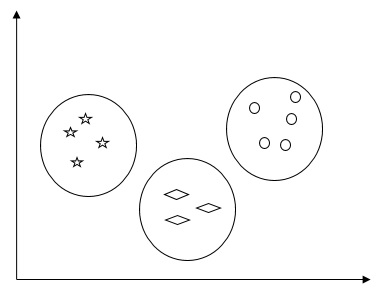### Algorithms for Clustering the Data

Following are a few common algorithms for clustering the data −

### K-Means algorithm

K-means clustering algorithm is one of the well-known algorithms for clustering the data. We need to assume that the numbers of clusters are already known. This is also called flat clustering. It is an iterative clustering algorithm. The steps given below need to be followed for this algorithm:

Step 1 − We need to specify the desired number of K subgroups.

Step 2 − Fix the number of clusters and randomly assign each data point to a cluster. Or in other words we need to classify our data based on the number of clusters.

In this step, cluster centroids should be computed.

As this is an iterative algorithm, we need to update the locations of K centroids with every iteration until we find the global optima or in other words the centroids reach at their optimal locations.

The following code will help in implementing K-means clustering algorithm in Python. We are going to use the Scikit-learn module.

[wpsbx_html_block id=1891]

Let us import the necessary packages −

import matplotlib.pyplot as plt
import seaborn as sns; sns.set()
import numpy as np
from sklearn.cluster import KMeans

The following line of code will help in generating the two-dimensional dataset, containing four blobs, by using make_blob from the sklearn.dataset package.

from sklearn.datasets.samples_generator import make_blobs

X, y_true = make_blobs(n_samples = 500, centers = 4,
cluster_std = 0.40, random_state = 0)

We can visualize the dataset by using the following code −

plt.scatter(X[:, 0], X[:, 1], s = 50);
plt.show()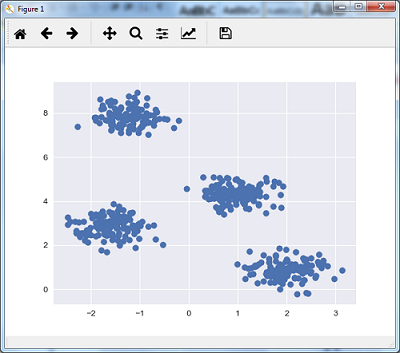Here, we are initializing kmeans to be the KMeans algorithm, with the required parameter of how many clusters (n_clusters).

kmeans = KMeans(n_clusters = 4)

We need to train the K-means model with the input data.

kmeans.fit(X)
y_kmeans = kmeans.predict(X)
plt.scatter(X[:, 0], X[:, 1], c = y_kmeans, s = 50, cmap = 'viridis')

centers = kmeans.cluster_centers_

The code given below will help us plot and visualize the machine’s findings based on our data, and the fitment according to the number of clusters that are to be found.

plt.scatter(centers[:, 0], centers[:, 1], c = 'black', s = 200, alpha = 0.5);
plt.show()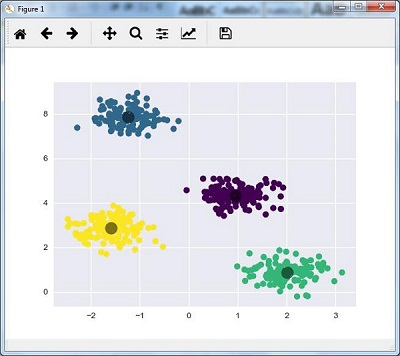### Mean Shift Algorithm

It is another popular and powerful clustering algorithm used in unsupervised learning. It does not make any assumptions hence it is a non-parametric algorithm. It is also called hierarchical clustering or mean shift cluster analysis. Followings would be the basic steps of this algorithm −

• First of all, we need to start with the data points assigned to a cluster of their own.
• Now, it computes the centroids and update the location of new centroids.
• By repeating this process, we move closer the peak of cluster i.e. towards the region of higher density.
• This algorithm stops at the stage where centroids do not move anymore.

With the help of following code we are implementing Mean Shift clustering algorithm in Python. We are going to use Scikit-learn module.

Let us import the necessary packages −

import numpy as np
from sklearn.cluster import MeanShift
import matplotlib.pyplot as plt
from matplotlib import style
style.use("ggplot")

The following code will help in generating the two-dimensional dataset, containing four blobs, by using make_blob from the sklearn.dataset package.

from sklearn.datasets.samples_generator import make_blobs

We can visualize the dataset with the following code

centers = [[2,2],[4,5],[3,10]]
X, _ = make_blobs(n_samples = 500, centers = centers, cluster_std = 1)
plt.scatter(X[:,0],X[:,1])
plt.show()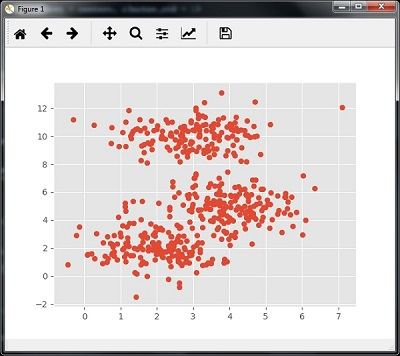Now, we need to train the Mean Shift cluster model with the input data.

ms = MeanShift()
ms.fit(X)
labels = ms.labels_
cluster_centers = ms.cluster_centers_

The following code will print the cluster centers and the expected number of cluster as per the input data −

print(cluster_centers)
n_clusters_ = len(np.unique(labels))
print("Estimated clusters:", n_clusters_)
[[ 3.23005036 3.84771893]
[ 3.02057451 9.88928991]]
Estimated clusters: 2

The code given below will help plot and visualize the machine’s findings based on our data, and the fitment according to the number of clusters that are to be found.

colors = 10*['r.','g.','b.','c.','k.','y.','m.']
for i in range(len(X)):
plt.plot(X[i], X[i], colors[labels[i]], markersize = 10)
plt.scatter(cluster_centers[:,0],cluster_centers[:,1],
marker = "x",color = 'k', s = 150, linewidths = 5, zorder = 10)
plt.show()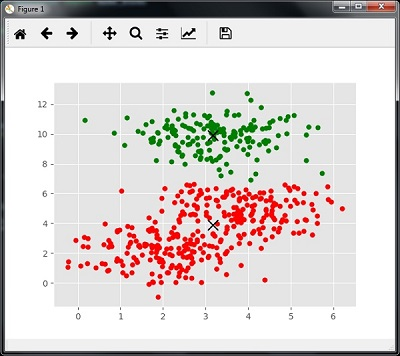### Measuring the Clustering Performance

The real world data is not naturally organized into number of distinctive clusters. Due to this reason, it is not easy to visualize and draw inferences. That is why we need to measure the clustering performance as well as its quality. It can be done with the help of silhouette analysis.

### Silhouette Analysis

This method can be used to check the quality of clustering by measuring the distance between the clusters. Basically, it provides a way to assess the parameters like number of clusters by giving a silhouette score. This score is a metric that measures how close each point in one cluster is to the points in the neighboring clusters.

### Analysis of silhouette score

The score has a range of [-1, 1]. Following is the analysis of this score −

• Score of +1 − Score near +1 indicates that the sample is far away from the neighboring cluster.
• Score of 0 − Score 0 indicates that the sample is on or very close to the decision boundary between two neighboring clusters.
• Score of -1 − Negative score indicates that the samples have been assigned to the wrong clusters.

### Calculating Silhouette Score

In this section, we will learn how to calculate the silhouette score.

Silhouette score can be calculated by using the following formula −

$$silhouette score = \frac{\left ( p-q \right )}{max\left ( p,q \right )}$$

Here, 𝑝 is the mean distance to the points in the nearest cluster that the data point is not a part of. And, 𝑞 is the mean intra-cluster distance to all the points in its own cluster.

For finding the optimal number of clusters, we need to run the clustering algorithm again by importing the metrics module from the sklearn package. In the following example, we will run the K-means clustering algorithm to find the optimal number of clusters −

Import the necessary packages as shown −

import matplotlib.pyplot as plt
import seaborn as sns; sns.set()
import numpy as np
from sklearn.cluster import KMeans

With the help of the following code, we will generate the two-dimensional dataset, containing four blobs, by using make_blob from the sklearn.dataset package.

from sklearn.datasets.samples_generator import make_blobs

X, y_true = make_blobs(n_samples = 500, centers = 4, cluster_std = 0.40, random_state = 0)

Initialize the variables as shown −

scores = []
values = np.arange(2, 10)

We need to iterate the K-means model through all the values and also need to train it with the input data.

for num_clusters in values:
kmeans = KMeans(init = 'k-means++', n_clusters = num_clusters, n_init = 10)
kmeans.fit(X)

Now, estimate the silhouette score for the current clustering model using the Euclidean distance metric −

score = metrics.silhouette_score(X, kmeans.labels_,
metric = 'euclidean', sample_size = len(X))

The following line of code will help in displaying the number of clusters as well as Silhouette score.

print("\nNumber of clusters =", num_clusters)
print("Silhouette score =", score)
scores.append(score)

You will receive the following output −

Number of clusters = 9
Silhouette score = 0.340391138371

num_clusters = np.argmax(scores) + values
print('\nOptimal number of clusters =', num_clusters)


Now, the output for optimal number of clusters would be as follows −

Optimal number of clusters = 2


### Finding Nearest Neighbors

The concept of finding nearest neighbors may be defined as the process of finding the closest point to the input point from the given dataset. The main use of this KNN)K-nearest neighbors) algorithm is to build classification systems that classify a data point on the proximity of the input data point to various classes.

The Python code given below helps in finding the K-nearest neighbors of a given data set −

Import the necessary packages as shown below. Here, we are using the NearestNeighbors module from the sklearn package

import numpy as np
import matplotlib.pyplot as plt
from sklearn.neighbors import NearestNeighbors

Let us now define the input data −

A = np.array([[3.1, 2.3], [2.3, 4.2], [3.9, 3.5], [3.7, 6.4], [4.8, 1.9],
[8.3, 3.1], [5.2, 7.5], [4.8, 4.7], [3.5, 5.1], [4.4, 2.9],])

Now, we need to define the nearest neighbors −

k = 3

We also need to give the test data from which the nearest neighbors is to be found −

test_data = [3.3, 2.9]

The following code can visualize and plot the input data defined by us −

plt.figure()
plt.title('Input data')
plt.scatter(A[:,0], A[:,1], marker = 'o', s = 100, color = 'black')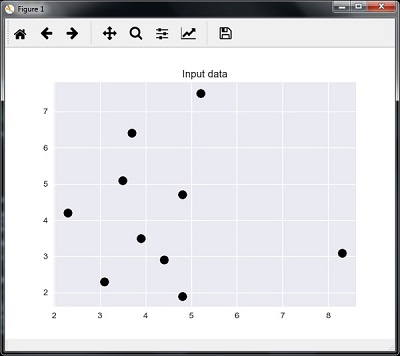Now, we need to build the K Nearest Neighbor. The object also needs to be trained

knn_model = NearestNeighbors(n_neighbors = k, algorithm = 'auto').fit(X)
distances, indices = knn_model.kneighbors([test_data])

Now, we can print the K nearest neighbors as follows

print("\nK Nearest Neighbors:")
for rank, index in enumerate(indices[:k], start = 1):
print(str(rank) + " is", A[index])

We can visualize the nearest neighbors along with the test data point

plt.figure()
plt.title('Nearest neighbors')
plt.scatter(A[:, 0], X[:, 1], marker = 'o', s = 100, color = 'k')
plt.scatter(A[indices][:][:, 0], A[indices][:][:, 1],
marker = 'o', s = 250, color = 'k', facecolors = 'none')
plt.scatter(test_data, test_data,
marker = 'x', s = 100, color = 'k')
plt.show()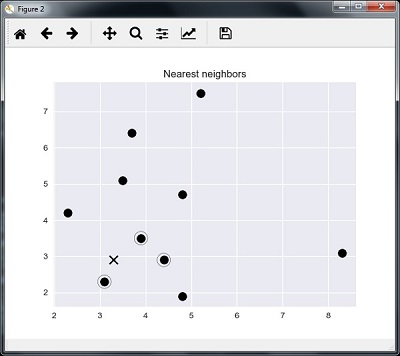### Output

K Nearest Neighbors

1 is [ 3.1 2.3]
2 is [ 3.9 3.5]
3 is [ 4.4 2.9]


### K-Nearest Neighbors Classifier

A K-Nearest Neighbors (KNN) classifier is a classification model that uses the nearest neighbors algorithm to classify a given data point. We have implemented the KNN algorithm in the last section, now we are going to build a KNN classifier using that algorithm.

### Concept of KNN Classifier

The basic concept of K-nearest neighbor classification is to find a predefined number, i.e., the ‘k’ − of training samples closest in distance to a new sample, which has to be classified. New samples will get their label from the neighbors itself. The KNN classifiers have a fixed user defined constant for the number of neighbors which have to be determined. For the distance, standard Euclidean distance is the most common choice. The KNN Classifier works directly on the learned samples rather than creating the rules for learning. The KNN algorithm is among the simplest of all machine learning algorithms. It has been quite successful in a large number of classification and regression problems, for example, character recognition or image analysis.

Example

We are building a KNN classifier to recognize digits. For this, we will use the MNIST dataset. We will write this code in the Jupyter Notebook.

Import the necessary packages as shown below.

Here we are using the KNeighborsClassifier module from the sklearn.neighbors package −

from sklearn.datasets import *
import pandas as pd
%matplotlib inline
from sklearn.neighbors import KNeighborsClassifier
import matplotlib.pyplot as plt
import numpy as np

The following code will display the image of digit to verify what image we have to test −

def Image_display(i):
plt.imshow(digit['images'][i],cmap = 'Greys_r')
plt.show()

Now, we need to load the MNIST dataset. Actually there are total 1797 images but we are using the first 1600 images as training sample and the remaining 197 would be kept for testing purpose.

digit = load_digits()
digit_d = pd.DataFrame(digit['data'][0:1600])

Now, on displaying the images we can see the output as follows −

Image_display(0)


### Image_display(0)

Image of 0 is displayed as follows −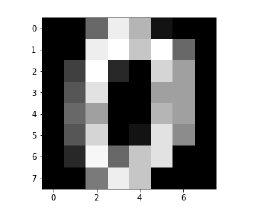### Image_display(9)

Image of 9 is displayed as follows −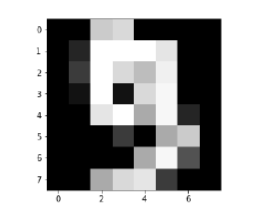### digit.keys()

Now, we need to create the training and testing data set and supply testing data set to the KNN classifiers.

train_x = digit['data'][:1600]
train_y = digit['target'][:1600]
KNN = KNeighborsClassifier(20)
KNN.fit(train_x,train_y)

The following output will create the K nearest neighbor classifier constructor −

KNeighborsClassifier(algorithm = 'auto', leaf_size = 30, metric = 'minkowski',
metric_params = None, n_jobs = 1, n_neighbors = 20, p = 2,
weights = 'uniform')


We need to create the testing sample by providing any arbitrary number greater than 1600, which were the training samples.

test = np.array(digit['data'])
test1 = test.reshape(1,-1)
Image_display(1725)

### Image_display(6)

Image of 6 is displayed as follows −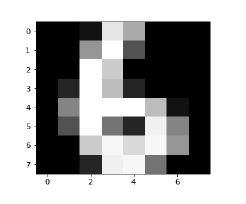Now we will predict the test data as follows −

KNN.predict(test1)

The above code will generate the following output −

array()


Now, consider the following −

digit['target_names']

The above code will generate the following output −

array([0, 1, 2, 3, 4, 5, 6, 7, 8, 9])## Supervised Learning: Regression

Regression is one of the most important statistical and machine learning tools. We would not be wrong to say that the journey of machine learning starts from regression. It may be defined as the parametric technique that allows us to make decisions based upon data or in other words allows us to make predictions based upon data by learning the relationship between input and output variables. Here, the output variables dependent on the input variables, are continuous-valued real numbers. In regression, the relationship between input and output variables matters and it helps us in understanding how the value of the output variable changes with the change of input variable. Regression is frequently used for prediction of prices, economics, variations, and so on.

### Building Regressors in Python

In this section, we will learn how to build single as well as multivariable regressor.

### Linear Regressor/Single Variable Regressor

Let us important a few required packages −

import numpy as np
from sklearn import linear_model
import sklearn.metrics as sm
import matplotlib.pyplot as plt

Now, we need to provide the input data and we have saved our data in the file named linear.txt.

input = 'D:/ProgramData/linear.txt'

input_data = np.loadtxt(input, delimiter=',')
X, y = input_data[:, :-1], input_data[:, -1]

The next step would be to train the model. Let us give training and testing samples.

training_samples = int(0.6 * len(X))
testing_samples = len(X) - num_training

X_train, y_train = X[:training_samples], y[:training_samples]

X_test, y_test = X[training_samples:], y[training_samples:]

Now, we need to create a linear regressor object.

reg_linear = linear_model.LinearRegression()

Train the object with the training samples.

reg_linear.fit(X_train, y_train)

We need to do the prediction with the testing data.

y_test_pred = reg_linear.predict(X_test)

Now plot and visualize the data.

plt.scatter(X_test, y_test, color = 'red')
plt.plot(X_test, y_test_pred, color = 'black', linewidth = 2)
plt.xticks(())
plt.yticks(())
plt.show()

### Output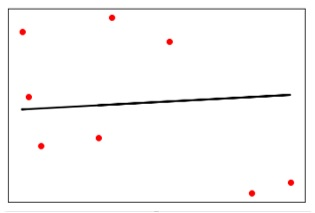Now, we can compute the performance of our linear regression as follows −

print("Performance of Linear regressor:")
print("Mean absolute error =", round(sm.mean_absolute_error(y_test, y_test_pred), 2))
print("Mean squared error =", round(sm.mean_squared_error(y_test, y_test_pred), 2))
print("Median absolute error =", round(sm.median_absolute_error(y_test, y_test_pred), 2))
print("Explain variance score =", round(sm.explained_variance_score(y_test, y_test_pred),
2))
print("R2 score =", round(sm.r2_score(y_test, y_test_pred), 2))

### Output

Performance of Linear Regressor −

Mean absolute error = 1.78
Mean squared error = 3.89
Median absolute error = 2.01
Explain variance score = -0.09
R2 score = -0.09


In the above code, we have used this small data. If you want some big dataset then you can use sklearn.dataset to import bigger dataset.

2,4.82.9,4.72.5,53.2,5.56,57.6,43.2,0.92.9,1.92.4,
3.50.5,3.41,40.9,5.91.2,2.583.2,5.65.1,1.54.5,
1.22.3,6.32.1,2.8

### Multivariable Regressor

First, let us import a few required packages −

import numpy as np
from sklearn import linear_model
import sklearn.metrics as sm
import matplotlib.pyplot as plt
from sklearn.preprocessing import PolynomialFeatures

Now, we need to provide the input data and we have saved our data in the file named linear.txt.

input = 'D:/ProgramData/Mul_linear.txt'

input_data = np.loadtxt(input, delimiter=',')
X, y = input_data[:, :-1], input_data[:, -1]

The next step would be to train the model; we will give training and testing samples.

training_samples = int(0.6 * len(X))
testing_samples = len(X) - num_training

X_train, y_train = X[:training_samples], y[:training_samples]

X_test, y_test = X[training_samples:], y[training_samples:]

Now, we need to create a linear regressor object.

reg_linear_mul = linear_model.LinearRegression()

Train the object with the training samples.

reg_linear_mul.fit(X_train, y_train)

Now, at last we need to do the prediction with the testing data.

y_test_pred = reg_linear_mul.predict(X_test)

print("Performance of Linear regressor:")
print("Mean absolute error =", round(sm.mean_absolute_error(y_test, y_test_pred), 2))
print("Mean squared error =", round(sm.mean_squared_error(y_test, y_test_pred), 2))
print("Median absolute error =", round(sm.median_absolute_error(y_test, y_test_pred), 2))
print("Explain variance score =", round(sm.explained_variance_score(y_test, y_test_pred), 2))
print("R2 score =", round(sm.r2_score(y_test, y_test_pred), 2))


### Output

Performance of Linear Regressor −

Mean absolute error = 0.6
Mean squared error = 0.65
Median absolute error = 0.41
Explain variance score = 0.34
R2 score = 0.33


Now, we will create a polynomial of degree 10 and train the regressor. We will provide the sample data point.

polynomial = PolynomialFeatures(degree = 10)
X_train_transformed = polynomial.fit_transform(X_train)
datapoint = [[2.23, 1.35, 1.12]]
poly_datapoint = polynomial.fit_transform(datapoint)

poly_linear_model = linear_model.LinearRegression()
poly_linear_model.fit(X_train_transformed, y_train)
print("\nLinear regression:\n", reg_linear_mul.predict(datapoint))
print("\nPolynomial regression:\n", poly_linear_model.predict(poly_datapoint))

### Output

Linear regression:

[2.40170462]


Polynomial regression:

[1.8697225]


In the above code, we have used this small data. If you want a big dataset then, you can use sklearn.dataset to import a bigger dataset.

2,4.8,1.2,3.22.9,4.7,1.5,3.62.5,5,2.8,23.2,5.5,3.5,2.16,5,
2,3.27.6,4,1.2,3.23.2,0.9,2.3,1.42.9,1.9,2.3,1.22.4,3.5,
2.8,3.60.5,3.4,1.8,2.91,4,3,2.50.9,5.9,5.6,0.81.2,2.58,
3.45,1.233.2,5.6,2,3.25.1,1.5,1.2,1.34.5,1.2,4.1,2.32.3,
6.3,2.5,3.22.1,2.8,1.2,3.6

[wpsbx_html_block id=1891]## Part 4: Artificial Intelligence on Natural Language Processing(NLP)

Natural Language Processing (NLP) refers to AI method of communicating with an intelligent systems using a natural language such as English. Processing of Natural Language is required when you want an intelligent system like robot to perform as per your instructions, when you want to hear decision from a dialogue based clinical expert system, etc. The field of NLP involves making computers to perform useful tasks with the natural languages humans use. The input and output of an NLP system can be −

• Speech
• Written Text

## Components of NLP

There are two components of NLP as given:

### Natural Language Understanding (NLU)

Understanding involves the following tasks −

• Mapping the given input in natural language into useful representations.
• Analyzing different aspects of the language.

### Natural Language Generation (NLG)

It is the process of producing meaningful phrases and sentences in the form of natural language from some internal representation.

It involves :

• Text planning − It includes retrieving the relevant content from knowledge base.
• Sentence planning − It includes choosing required words, forming meaningful phrases, setting tone of the sentence.
• Text Realization − It is mapping sentence plan into sentence structure.

The NLU is harder than NLG.

### Difficulties in NLU

NLP has an extremely rich form and structure. It is very ambiguous. There can be different levels of ambiguity −

• Lexical ambiguity − It is at very primitive level such as word-level.
• For example, treating the word “board” as noun or verb?
• Syntax Level ambiguity − A sentence can be parsed in different ways.
• For example, “He lifted the beetle with red cap.” − Did he use cap to lift the beetle or he lifted a beetle that had red cap?
• Referential ambiguity − Referring to something using pronouns. For example, Rima went to Gauri. She said, “I am tired.” − Exactly who is tired?
• One input can mean different meanings.
• Many inputs can mean the same thing.

### NLP Terminology

• Phonology − It is study of organizing sound systematically.
• Morphology − It is a study of construction of words from primitive meaningful units.
• Morpheme − It is primitive unit of meaning in a language.
• Syntax − It refers to arranging words to make a sentence. It also involves determining the structural role of words in the sentence and in phrases.
• Semantics − It is concerned with the meaning of words and how to combine words into meaningful phrases and sentences.
• Pragmatics − It deals with using and understanding sentences in different situations and how the interpretation of the sentence is affected.
• Discourse − It deals with how the immediately preceding sentence can affect the interpretation of the next sentence.
• World Knowledge − It includes the general knowledge about the world.

[wpsbx_html_block id=1891]

### Steps in NLP

There are general five(5) steps:

• Lexical Analysis − It involves identifying and analyzing the structure of words. Lexicon of a language means the collection of words and phrases in a language. Lexical analysis is dividing the whole chunk of txt into paragraphs, sentences,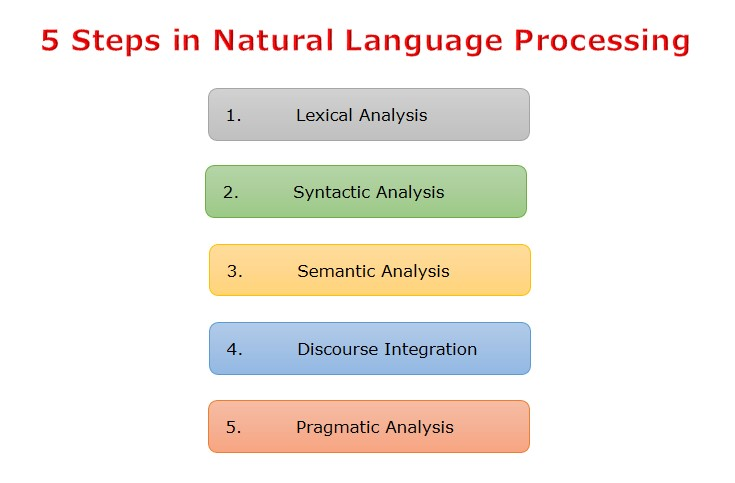and words.
• Syntactic Analysis (Parsing) − It involves analysis of words in the sentence for grammar and arranging word
• s in a manner that shows the relationship among the words. The sentence such as “The school goes to boy” is rejected by English syntactic analyzer.
• Semantic Analysis − It draws the exact meaning or the dictionary meaning from the text. The text
is checked for meaningfulness. It is done by mapping syntactic structures and objects in the task domain. The semantic analyzer disregards sentence such as “hot ice-cream”.
• Discourse Integration − The meaning of any sentence depends upon the meaning of the sentence just before it. In addition, it also brings about the meaning of immediately succeeding sentence.
• Pragmatic Analysis − During this, what was said is re-interpreted on what it actually meant. It involves deriving those aspects of language which require real world knowledge.

### Implementation Aspects of Syntactic Analysis

There are a number of algorithms researchers have developed for syntactic analysis, but we consider only the following simple methods:

• Context-Free Grammar
• Top-Down Parser

Let us see them in detail:

### Context-Free Grammar

It is the grammar that consists rules with a single symbol on the left-hand side of the rewrite rules. Let us create grammar to parse a sentence:

“The bird pecks the grains”

Articles (DET) − a | an | the

Nouns − bird | birds | grain | grains

Noun Phrase (NP) − Article + Noun | Article + Adjective + Noun

= DET N | DET ADJ N

Verbs − pecks | pecking | pecked

Verb Phrase (VP) − NP V | V NP

The parse tree breaks down the sentence into structured parts so that the computer can easily understand and process it. In order for the parsing algorithm to construct this parse tree, a set of rewrite rules, which describe what tree structures are legal, need to be constructed. These rules say that a certain symbol may be expanded in the tree by a sequence of other symbols. According to first order logic rule, if there are two strings Noun Phrase (NP) and Verb Phrase (VP), then the string combined by NP followed by VP is a sentence. The rewrite rules for the sentence are as follows −

S → NP VP

NP → DET N | DET ADJ N

VP → V NP

Lexocon −

DET → a | the

N → bird | birds | grain | grains

V → peck | pecks | pecking

The parse tree can be created as shown −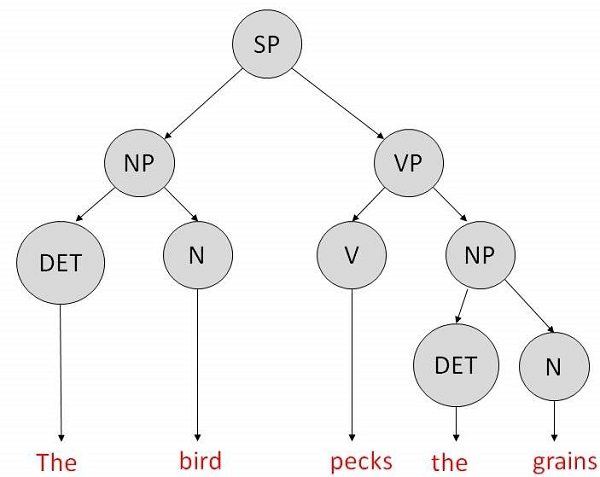Now consider the above rewrite rules. Since V can be replaced by both, “peck” or “pecks”, sentences such as “The bird peck the grains” can be wrongly permitted. i. e. the subject-verb agreement error is approved as correct.

Merit:The simplest style of grammar, therefore widely used one.

Demerits:

• They are not highly precise. For example, “The grains peck the bird”, is a syntactically correct according to parser, but even if it makes no sense, parser takes it as a correct sentence.
• To bring out high precision, multiple sets of grammar need to be prepared. It may require a completely different sets of rules for parsing singular and plural variations, passive sentences, etc., which can lead to creation of huge set of rules that are unmanageable.

### Top-Down Parser

Here, the parser starts with the S symbol and attempts to rewrite it into a sequence of terminal symbols that matches the classes of the words in the input sentence until it consists entirely of terminal symbols. These are then checked with the input sentence to see if it matched. If not, the process is started over again with a different set of rules. This is repeated until a specific rule is found which describes the structure of the sentence.

Merit: It is simple to implement.

Demerits:

• It is inefficient, as the search process has to be repeated if an error occurs.
• Slow speed of working.## Part 3: Artificial Intelligence Popular Search Algorithms

Searching is the universal technique of problem solving in AI. There are some single-player games such as tile games, Sudoku, crossword, etc. The search algorithms help you to search for a particular position in such games.

## Single Agent Path finding Problems

The games such as 3X3 eight-tile, 4X4 fifteen-tile, and 5X5 twenty four tile puzzles are single-agent-path-finding challenges. They consist of a matrix of tiles with a blank tile. The player is required to arrange the tiles by sliding a tile either vertically or horizontally into a blank space with the aim of accomplishing some objective. The other examples of single agent pathfinding problems are Travelling Salesman Problem, Rubik’s Cube, and Theorem Proving.

## Search Terminology

• Problem Space: It is the environment in which the search takes place. (A set of states and set of operators to change those states)
• Problem Instance : It is Initial state + Goal state.
• Problem Space Graph: It represents problem state. States are shown by nodes and operators are shown by edges.
• Depth of a problem: Length of a shortest path or shortest sequence of operators from Initial State to goal state.
• Space Complexity: The maximum number of nodes that are stored in memory.
• Time Complexity: The maximum number of nodes that are created.
• Admissibility: A property of an algorithm to always find an optimal solution.
• Branching Factor: The average number of child nodes in the problem space graph.
• Depth: Length of the shortest path from initial state to goal state.

### Brute-Force Search Strategies

They are most simple, as they do not need any domain-specific knowledge. They work fine with small number of possible states. Requirements:

• State description
• A set of valid operators
• Initial state
• Goal state description

It starts from the root node, explores the neighboring nodes first and moves towards the next level neighbors. It generates one tree at a time until the solution is found. It can be implemented using FIFO queue data structure. This method provides shortest path to the solution. If branching factor (average number of child nodes for a given node) = b and depth = d, then number of nodes at level d = bd.

The total no of nodes created in worst case is b + b2 + b3 + … + bd.

Disadvantage − Since each level of nodes is saved for creating next one, it consumes a lot of memory space. Space requirement to store nodes is exponential.

Its complexity depends on the number of nodes. It can check duplicate nodes.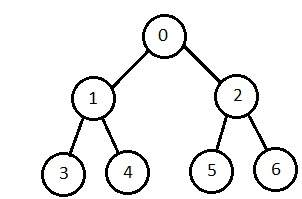### Depth-First Search(DFS)

It is implemented in recursion with LIFO stack data structure. It creates the same set of nodes as Breadth-First method, only in the different order. As the nodes on the single path are stored in each iteration from root to leaf node, the space requirement to store nodes is linear. With branching factor b and depth as m, the storage space is bm.

Disadvantage − This algorithm may not terminate and go on infinitely on one path. The solution to this issue is to choose a cut-off depth. If the ideal cut-off is d, and if chosen cut-off is lesser than d, then this algorithm may fail. If chosen cut-off is more than d, then execution time increases.

Its complexity depends on the number of paths. It cannot check duplicate nodes.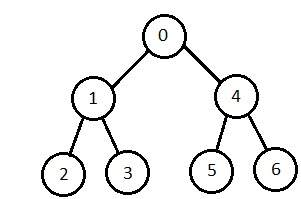### Bidirectional Search

It searches forward from initial state and backward from goal state till both meet to identify a common state. The path from initial state is concatenated with the inverse path from the goal state. Each search is done only up to half of the total path.

[wpsbx_html_block id=1891]

### Uniform Cost Search

Sorting is done in increasing cost of the path to a node. It always expands the least cost node. It is identical to Breadth First search if each transition has the same cost. It explores paths in the increasing order of cost.

Disadvantage − There can be multiple long paths with the cost ≤ C*. Uniform Cost search must explore them all.

### Iterative Deepening Depth-First Search

It performs depth-first search to level 1, starts over, executes a complete depth-first search to level 2, and continues in such way till the solution is found. It never creates a node until all lower nodes are generated. It only saves a stack of nodes. The algorithm ends when it finds a solution at depth d. The number of nodes created at depth d is bd and at depth d-1 is bd-1.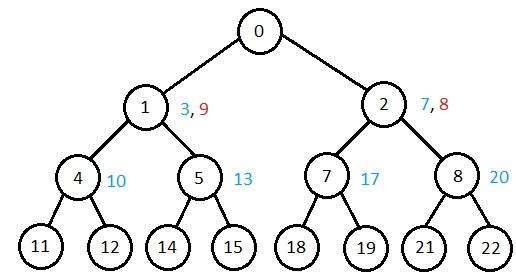### Comparison of Various Algorithms Complexities

Let us see the performance of algorithms based on various criteria:

Criterion Breadth First Depth First Bidirectional Uniform Cost Interactive Deepening
Time bd bm bd/2 bd bd
Space bd bm bd/2 bd bd
Optimality Yes No Yes Yes Yes
Completeness Yes No Yes Yes Yes

### Informed (Heuristic) Search Strategies

To solve large problems with large number of possible states, problem-specific knowledge needs to be added to increase the efficiency of search algorithms.

### Heuristic Evaluation Functions

They calculate the cost of optimal path between two states. A heuristic function for sliding-tiles games is computed by counting number of moves that each tile makes from its goal state and adding these number of moves for all tiles.

### Pure Heuristic Search

It expands nodes in the order of their heuristic values. It creates two lists, a closed list for the already expanded nodes and an open list for the created but unexpanded nodes. In each iteration, a node with a minimum heuristic value is expanded, all its child nodes are created and placed in the closed list. Then, the heuristic function is applied to the child nodes and they are placed in the open list according to their heuristic value. The shorter paths are saved and the longer ones are disposed.

### A * Search

It is best-known form of Best First search. It avoids expanding paths that are already expensive, but expands most promising paths first.

f(n) = g(n) + h(n), where

• g(n) the cost (so far) to reach the node
• h(n) estimated cost to get from the node to the goal
• f(n) estimated total cost of path through n to goal. It is implemented using priority queue by increasing f(n).

### Greedy Best First Search

It expands the node that is estimated to be closest to goal. It expands nodes based on f(n) = h(n). It is implemented using priority queue.

Disadvantage − It can get stuck in loops. It is not optimal.

### Local Search Algorithms

They start from a prospective solution and then move to a neighboring solution. They can return a valid solution even if it is interrupted at any time before they end.

### Hill-Climbing Search

It is an iterative algorithm that starts with an arbitrary solution to a problem and attempts to find a better solution by changing a single element of the solution incrementally. If the change produces a better solution, an incremental change is taken as a new solution. This process is repeated until there are no further improvements.

function Hill-Climbing (problem), returns a state that is a local maximum.

inputs: problem, a problem
local variables: current, a node
neighbor, a node
current <-Make_Node(Initial-State[problem])
loop
do neighbor <- a highest_valued successor of current
if Value[neighbor] ≤ Value[current] then
return State[current]
current <- neighbor

end


Disadvantage − This algorithm is neither complete, nor optimal.

### Local Beam Search

In this algorithm, it holds k number of states at any given time. At the start, these states are generated randomly. The successors of these k states are computed with the help of objective function. If any of these successors is the maximum value of the objective function, then the algorithm stops. Otherwise the (initial k states and k number of successors of the states = 2k) states are placed in a pool. The pool is then sorted numerically. The highest k states are selected as new initial states. This process continues until a maximum value is reached.

function BeamSearch( problem, k), returns a solution state.

start with k randomly generated states
loop
generate all successors of all k states
if any of the states = solution, then return the state
else select the k best successors
end


### Simulated Annealing

Annealing is the process of heating and cooling a metal to change its internal structure for modifying its physical properties. When the metal cools, its new structure is seized, and the metal retains its newly obtained properties. In simulated annealing process, the temperature is kept variable. We initially set the temperature high and then allow it to ‘cool’ slowly as the algorithm proceeds. When the temperature is high, the algorithm is allowed to accept worse solutions with high frequency.

Start

• Initialize k = 0; L = integer number of variables;
• From i → j, search the performance difference Δ.
• If Δ <= 0 then accept else if exp(-Δ/T(k)) > random(0,1) then accept;
• Repeat steps 1 and 2 for L(k) steps.
• k = k + 1;

Repeat steps 1 through 4 till the criteria is met.

End

### Travelling Salesman Problem

In this algorithm, the objective is to find a low-cost tour that starts from a city, visits all cities en-route exactly once and ends at the same starting city.

Start
Find out all (n -1)! Possible solutions, where n is the total number of cities.
Determine the minimum cost by finding out the cost of each of these (n -1)! solutions.
Finally, keep the one with the minimum cost.
end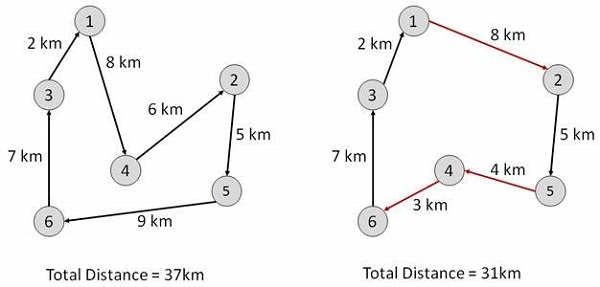## What is Artificial Intelligence?

AI has the potential to help humans live more meaningful lives that are devoid of hard labour. According to the father of Artificial Intelligence, John McCarthy, it is “The science and engineering of making intelligent machines, especially intelligent computer programs”.

Artificial Intelligence is a way of making a computer, a computer-controlled robot, or a software think intelligently, in the similar manner the intelligent humans think. AI is accomplished by studying how human brain thinks, and how humans learn, decide, and work while trying to solve a problem, and then using the outcomes of this study as a basis of developing intelligent software and systems.

### Goals of AI

• To Create Expert Systems − The systems which exhibit intelligent behavior, learn, demonstrate, explain, and advice its users.
• To Implement Human Intelligence in Machines − Creating systems that understand, think, learn, and behave like humans.

### Programming Without and With AI

The programming without and with AI is different in following ways −

Programming Without AI Programming With AI
A computer program without AI can answer the specific questions it is meant to solve. A computer program with AI can answer the generic questions it is meant to solve.
Modification in the program leads to change in its structure. AI programs can absorb new modifications by putting highly independent pieces of information together. Hence you can modify even a minute piece of information of program without affecting its structure.
Modification is not quick and easy. It may lead to affecting the program adversely. Quick and Easy program modification.

### What is AI Technique?

In the real world, the knowledge has some unwelcomed properties:

• Its volume is huge, next to unimaginable.
• It is not well-organized or well-formatted.
• It keeps changing constantly.

AI Technique is a manner to organize and use the knowledge efficiently in such a way that:

• It should be perceivable by the people who provide it.
• It should be easily modifiable to correct errors.
• It should be useful in many situations though it is incomplete or inaccurate.

AI techniques elevate the speed of execution of the complex program it is equipped with.

### Applications of AI

AI has been dominant in various fields such as:

• Gaming − AI plays crucial role in strategic games such as chess, poker, tic-tac-toe, etc., where machine can think of large number of possible positions based on heuristic knowledge.
• Natural Language Processing − It is possible to interact with the computer that understands natural language spoken by humans.
• Expert Systems − There are some applications which integrate machine, software, and special information to impart reasoning and advising. They provide explanation and advice to the users.
• Vision Systems − These systems understand, interpret, and comprehend visual input on the computer. For example,
• A spying aeroplane takes photographs, which are used to figure out spatial information or map of the areas.
• Doctors use clinical expert system to diagnose the patient.
• Police use computer software that can recognize the face of criminal with the stored portrait made by forensic artist.
• Speech Recognition − Some intelligent systems are capable of hearing and comprehending the language in terms of sentences and their meanings while a human talks to it. It can handle different accents, slang words, noise in the background, change in human’s noise due to cold, etc.
• Handwriting Recognition − The handwriting recognition software reads the text written on paper by a pen or on screen by a stylus. It can recognize the shapes of the letters and convert it into editable text.
• Intelligent Robots − Robots are able to perform the tasks given by a human. They have sensors to detect physical data from the real world such as light, heat, temperature, movement, sound, bump, and pressure. They have efficient processors, multiple sensors and huge memory, to exhibit intelligence. In addition, they are capable of learning from their mistakes and they can adapt to the new environment.

[wpsbx_html_block id=1891]

Following are some main advantages of Artificial Intelligence:

• High Accuracy with less errors: AI machines or systems are prone to less errors and high accuracy as it takes decisions as per pre-experience or information.
• High-Speed: AI systems can be of very high-speed and fast-decision making, because of that AI systems can beat a chess champion in the Chess game.
• High reliability: AI machines are highly reliable and can perform the same action multiple times with high accuracy.
• Useful for risky areas: AI machines can be helpful in situations such as defusing a bomb, exploring the ocean floor, where to employ a human can be risky.
• Digital Assistant: AI can be very useful to provide digital assistant to the users such as AI technology is currently used by various E-commerce websites to show the products as per customer requirement.
• Useful as a public utility: AI can be very useful for public utilities such as a self-driving car which can make our journey safer and hassle-free, facial recognition for security purpose, Natural language processing to communicate with the human in human-language, etc.

Every technology has some disadvantages, and thesame goes for Artificial intelligence. Being so advantageous technology still, it has some disadvantages which we need to keep in our mind while creating an AI system. Following are the disadvantages of AI:

• High Cost: The hardware and software requirement of AI is very costly as it requires lots of maintenance to meet current world requirements.
• Can’t think out of the box: Even we are making smarter machines with AI, but still they cannot work out of the box, as the robot will only do that work for which they are trained, or programmed.
• No feelings and emotions: AI machines can be an outstanding performer, but still it does not have the feeling so it cannot make any kind of emotional attachment with human, and may sometime be harmful for users if the proper care is not taken.
• Increase dependency on machines: With the increment of technology, people are getting more dependent on devices and hence they are losing their mental capabilities.
• No Original Creativity: As humans are so creative and can imagine some new ideas but still AI machines cannot beat this power of human intelligence and cannot be creative and imaginative.

### What is Intelligence?

The ability of a system to calculate, reason, perceive relationships and analogies, learn from experience, store and retrieve information from memory, solve problems, comprehend complex ideas, use natural language fluently, classify, generalize, and adapt new situations.

### What is Intelligence Composed of?

The intelligence is intangible. It is composed of −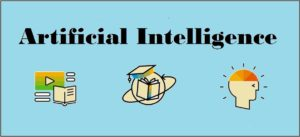• Reasoning
• Learning
• Problem Solving
• Perception
• Linguistic Intelligence

Let us go through all the components briefly −

• Reasoning − It is the set of processes that enables us to provide basis for judgement, making decisions, and prediction. There are broadly two types −
Inductive Reasoning Deductive Reasoning
It conducts specific observations to makes broad general statements. It starts with a general statement and examines the possibilities to reach a specific, logical conclusion.
Even if all of the premises are true in a statement, inductive reasoning allows for the conclusion to be false. If something is true of a class of things in general, it is also true for all members of that class.
Example − “Nita is a teacher. Nita is studious. Therefore, All teachers are studious.” Example − “All women of age above 60 years are grandmothers. Shalini is 65 years. Therefore, Shalini is a grandmother.”
• Learning − It is the activity of gaining knowledge or skill by studying, practising, being taught, or experiencing something. Learning enhances the awareness of the subjects of the study.The ability of learning is possessed by humans, some animals, and AI-enabled systems. Learning is categorized as −
• Auditory Learning − It is learning by listening and hearing. For example, students listening to recorded audio lectures.
• Episodic Learning − To learn by remembering sequences of events that one has witnessed or experienced. This is linear and orderly.
• Motor Learning − It is learning by precise movement of muscles. For example, picking objects, Writing, etc.
• Observational Learning − To learn by watching and imitating others. For example, child tries to learn by mimicking her parent.
• Perceptual Learning − It is learning to recognize stimuli that one has seen before. For example, identifying and classifying objects and situations.
• Relational Learning − It involves learning to differentiate among various stimuli on the basis of relational properties, rather than absolute properties. For Example, Adding ‘little less’ salt at the time of cooking potatoes that came up salty last time, when cooked with adding say a tablespoon of salt.
• Spatial Learning − It is learning through visual stimuli such as images, colors, maps, etc. For Example, A person can create roadmap in mind before actually following the road.
• Stimulus-Response Learning − It is learning to perform a particular behavior when a certain stimulus is present. For example, a dog raises its ear on hearing doorbell.
• Problem Solving − It is the process in which one perceives and tries to arrive at a desired solution from a present situation by taking some path, which is blocked by known or unknown hurdles.Problem solving also includes decision making, which is the process of selecting the best suitable alternative out of multiple alternatives to reach the desired goal are available.
• Perception − It is the process of acquiring, interpreting, selecting, and organizing sensory information.Perception presumes sensing. In humans, perception is aided by sensory organs. In the domain of AI, perception mechanism puts the data acquired by the sensors together in a meaningful manner.
• Linguistic Intelligence − It is one’s ability to use, comprehend, speak, and write the verbal and written language. It is important in interpersonal communication.

### Difference between Human and Machine Intelligence

• Humans perceive by patterns whereas the machines perceive by set of rules and data.
• Humans store and recall information by patterns, machines do it by searching algorithms. For example, the number 40404040 is easy to remember, store, and recall as its pattern is simple.
• Humans can figure out the complete object even if some part of it is missing or distorted; whereas the machines cannot do it correctly.